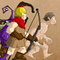...    players online request your hacks

Age of Defense 4 HackedName:
Age of Defense 4
Rate:
(Avg. 4.55) ...
Categories:
Strategy Upgrade Belugerin Click to find more games like this.

Game Description

Age of Defense 4 is a mix between defense and strategy warfare game, unleash unit, destroy enemy defense and defend your base with ultimate weapons!

How To Play

Move mouse to the edge of screen to scroll map or use LEFT/RIGHT and A/D.
Click any unit’s icons below to unleash units.
Hold mouse to increase the throw power, then release it to throw a thrown object.
Press mouse to shoot arrows and guns, when ammo empty, it will automatically reload.
Press 1 to use primary weapon.
Press 2 to use secondary weapon.
Press Space Bar to cast special weapons.
Click world to set background.
When blue bar maximum. weapons will affect special effects: fire, poison, explode and light.
Click Spell Button to use spell, each age own different spell.
Press P or click menu to pause.
See in-game instructions for further information.

Feedback for Age of Defense 4

• 4th this is my first comment ever just made an account
• and its 3rd not 3st you dumbass
• 1st :D Hey i never knew there was a part 4!
• YeS Its finally out !!!!!! yes! yes yes! YES!
• wow cooooooooooooooooooooooooooool
• scroll down

fun

**** you dieeeeeeeeeeeeeeeeeeeeeeeeee
• scroll down
.
.
.
.
.
.
.
.
.
.
.
.
.
.
.
Scroll up
.
.
.
.
.
.
.
.
.
.
.
.
.
.
i said scroll up!!!
.
.
.
.
.
.
.
.
.
.
.
.
.
.
.
.
it's danger
.
.
.
.
.
.
.
.
there was a ghost in your back !!!!!
• lolololololollolololololololollolololollololollolollol^lololololollloolololololololololololololololloololololololololololololololololololololololloloolololololloolololloolololololololololloloolollol|
• LOL @Xenon what program you used to make this animation?
• www.animateit.net
• go down # ^^^^^^^^^^^^^^^^^^^^^^^^^^^^^^^^^^^^^^^^^^^^^^^^^^^^^^^^^^^^^^^^^^^^^^^^^^^^^^^^^^^^^^^^^^^^^^^^^^^^^^^^^^^^^^^^^^^^^^^^^^^^^^^^^^^^^^^^^^^^^^^^^^^^^^^^^^^^^^^^^^^^^^^^^^^^^^^^^^^^^^^^^^^^^^^^^^^^^^^^^^^^^^^^^^^^^^^^^^^^^^^^^^^^^^^^^^^^^^^^^^^^^^^^^^^^^^^^^^^^^^^^^^^^^^^^^^^^^^^^^^^^^^^^^^^^^^^^^^^^^^^^^^^^^^^^^^^^^^^^^^^^^^^^^^^^^^^^^^^^^^^^^^^^^^^^^^^^^^^^^^^^^^^^^^^^^^^^^^^^^^^^^^^^^^^^^^^^^^^^^^^^^^^^^^^^^^^^^^^^^^^^^^^^^^^^^^^^^^^^^^^^^^^^^^^^^^^^^^^^^^^^^^^^^^^^^^^^^^^^^^^^^^^^^^^^^^^^^^^^^^^^^^^^^^^^^^^^^^^^^^^^^^^^^^^^^^^^^^^^^^^^^^^^^^^^^^^^^^^^^^^^^^^^^^^^^^^^^^^^^^^^^^^^^^^^^^^^^^^^^^^^^^^^^^^^^^^^^^^^^^^^^^^^^^^^^^^^^^^^^ you will die